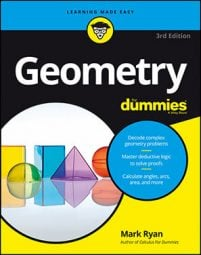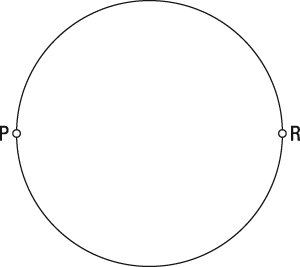##### Geometry For DummiesIf you're given two points on the coordinate plane, you can find the locus of points that creates a right angle to those two points. By using the following steps, you'll soon see an interesting pattern that may, in fact, surprise you.

Here's a problem to solve: Given points P and R, what's the locus of points Q such that angle PQR is a right angle?

1. Identify a pattern. This pattern may be a bit tricky to find, but if you start with points P and R and try to find a few points Q that make a right angle with P and R, you'll probably begin to see a pattern emerging, as shown here.

See the pattern? The Q points are beginning to form a circle with diameter PR. This makes sense if you think about the inscribed-angle theorem: In a circle with line PR as its diameter, semicircular arc PR would be 180 degrees, so all inscribed angles PQR would be one-half of that, or 90 degrees.

2. Look outside the pattern. Nope, nothing to add here. Any point Q inside the circle you identified in Step 1 creates an obtuse angle with P and R (or a straight angle), and any point Q outside the circle creates an acute angle with P and R (or a zero degree angle). All the right angles are on the circle.
3. Look inside the pattern. Bingo. See what points have to be excluded? It's the given points P and R. If Q is at the location of either given point, all you have left is a segment (QR or PQ), so you no longer have the three distinct points you need to make an angle.
4. Draw the locus and describe it in words.Given points P and R, the locus of points Q such that angle PQR is a right angle is a circle with diameter PR, minus points P and R.

The final figure shows the locus, and the caption gives its description. Note the hollow dots at P and R, which indicate that those points aren't part of the solution.